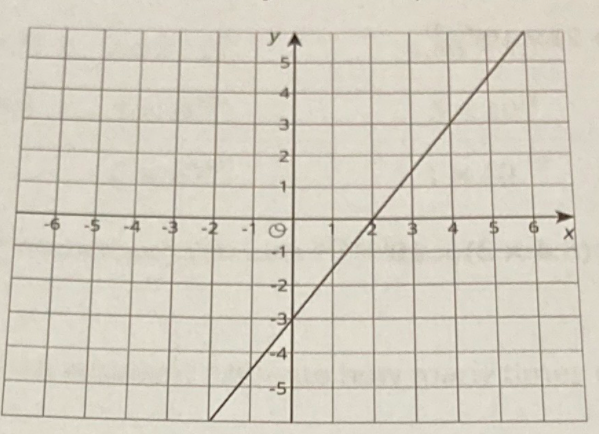### Still have math questions?

Algebra
Question

Here is the graph for one equation in a system of equations.a. Write a second equation for the system so it has infinitely many solutions.

b. Write a second equation whose graph goes through $$( 0,2 )$$ so that the system

has no solutions.

c. Write a second equation whose graph goes through $$( 2,2 )$$ so that the system

has one solution at $$( 4,3 )$$ .

a.$$y= 1.5x- 3$$
b.$$y= 1.5x+ 2$$
c.$$y= 0.5x+ 1$$